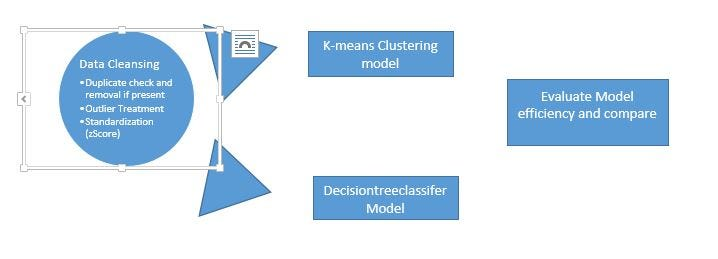# Unsupervised vs. Supervised LearningI just started my initial steps into data science and machine learning, and, got introduced to “Supervised Learning” techniques as “Classifiers (Decisiontreeclassifer from sklearn kit).

I just started my initial steps into data science and machine learning, and, got introduced to “Supervised Learning” techniques as “Classifiers (Decisiontreeclassifer from sklearn kit), and on the unsupervised learning, with “Clustering.”

In this case, we are using the dataset “Breast cancer — Wisconsin” and set the following objective:

*a) *Perform clustering (k-means), use evaluation methods like silhouette score and WSS (within the sum of squares) to find optimal clusters,

b) Perform a Decisiontreeclassifier model, and the traditional train versus test samples and evaluate the model with ROC/AUC

*c) *Compare the clustering model output with the efficiency of Decisiontreeclassifer model outcome

The comparison outcome, presented a surprise to me, were without the target/class variables, the accuracy with just clustering, was close to 95 % match to the actual class variables in the data set, better than Supervised learning (with 70: 30, train to test split up, the accuracy was 92 % ). Now, does this mean it will work for larger samples also, is to be validated for larger data sets?

Let us get started — Data insights :

Features are a digitized image compilation of a fine needle aspirate (FNA) of a breast mass. They describe the characteristics of the cell nuclei present in the image.

Total rows — 569, columns — 32 (including class variable, called diagnosis, with the outcome as Malignant (M) and Benign (B).

## Decision Trees Classifier

Decision Trees Classifier - Both of Regression Trees and Classification Trees are a part of CART (Classification And Regression Tree) Algorithm.

## Decision Tree -Classification

Decision Tree is one of the most widely used machine learning algorithm. It is a supervised learning algorithm that can perform both classification and regression operations.

## Maths Behind Decision Tree Classifier

Maths behind Decision Tree Classifier. Before we see the python implementation of the decision tree. Let’s first understand the math behind the decision tree classification.

## PyTorch For Deep Learning — Confusion Matrix

This is a regular classification problem with PyTorch and this is exactly like the one in the previous post of the “PyTorch for Deep Learning” series. The Reason for doing writing the post is for some more reference to classification problem and better understanding.

## Supervised Learning vs Unsupervised Learning

Supervised Learning vs Unsupervised Learning. This article will introduce us to the tools and techniques developed to make sense of unstructured data and discover hidden patterns.